## Analysis and Partial Differential Equations

### Analysis and Partial Differential Equations

The research interests of the Analysis Group lie mainly in functional analysis, complex analysis and in the analysis of partial differential equations. On the functional and complex analysis part the structure theory of locally convex spaces including spaces of analytic, harmonic, and infinitely differentiable functions of several variables is studied whereas in the partial differential equations part some applications to nonlinear mechanics is also considered.

The following areas are of particular interest: linear topological invariants, isomorphisms and bases in locally convex spaces; complex potential theory, approximation and interpolation of analytic and harmonic functions, composition operators on analytic function spaces; probability measures in infinite dimensional spaces, nonlinear theory of distributions; as well as operator theory, pseudo-differential operators, nonlinear partial differential equations, infinite-dimensional dynamical systems, calculus of variations and their applications to mechanical problems. In this mathematical landscape, geometry, functional analysis, complex and real analysis work together to create a rich set of phenomena, many of which still have to be understood.

Group members take part in the Istanbul Analysis Seminars and Istanbul Differential Equations Seminars.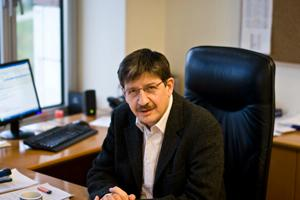Albert Erkip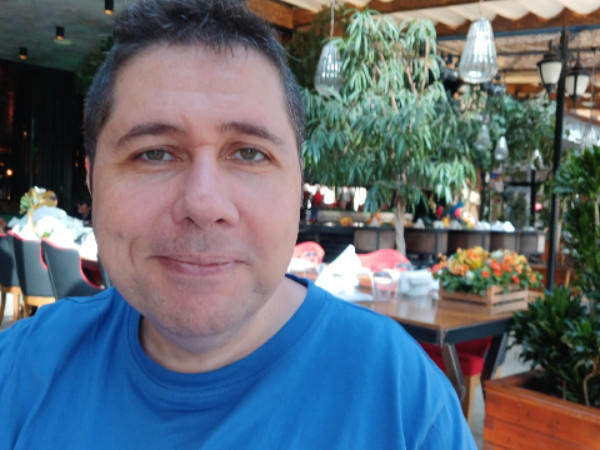Nihat Gökhan Göğüş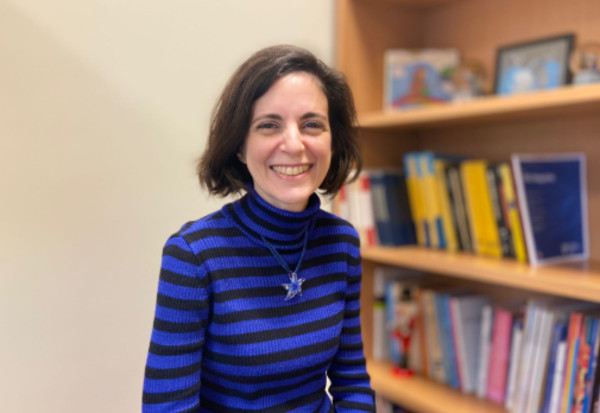Nilay Duruk Mutlubaş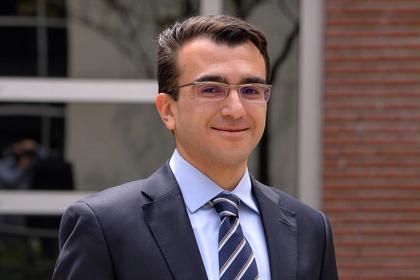Semih Onur SezerTurgay Bayraktar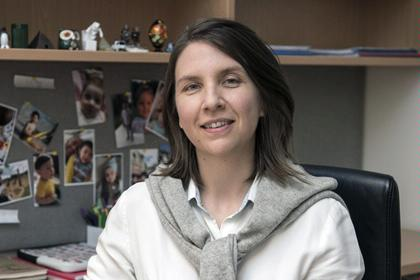Yasemin Şengül Tezel